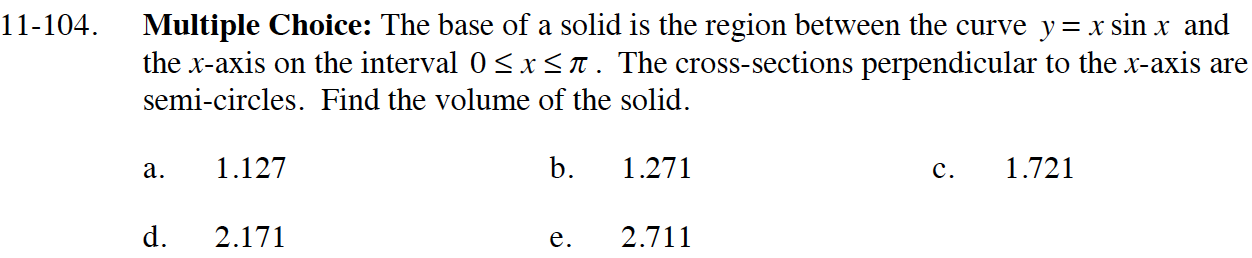### Home > CALC > Chapter 11 > Lesson 11.3.1 > Problem11-104

11-104.
1. Multiple Choice: The base of a solid is the region between the curve y = x sin x and the x-axis on the interval 0 ≤ xπ. The cross-sections perpendicular to the x-axis are semi-circles. Find the volume of the solid. Homework Help ✎

1. 1.127

2. 1.271

3. 1.721

4. 2.171

5. 2.711The diameter of each semicircle is y/2.
The area of each semicircle is π(r2)/2.
The width of each cross-section is dx.